Pure and Applied Mathematics Journal
Volume 5, Issue 5, October 2016, Pages: 151-154

An Application of the New Integral "Aboodh Transform" in Cryptography

Abdelilah K. Hassan Sedeeg1, 2, Mohand M. Abdelrahim Mahgoub1, 3, Muneer A. Saif Saeed4

1Mathematics Department Faculty of Sciences and Arts, Almikwah-Albaha University, Albaha, Saudi Arabia

2Mathematics Department Faculty of Education, Holy Quran and Islamic Sciences University, Khartoum, Sudan

3Mathematics Department Faculty of Sciences, Omdurman Islamic University, Khartoum, Sudan

4Computer Department Faculty of Sciences and Arts, Almikwah-Albaha University, Albaha, Saudi Arabia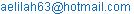(A. K. H. Sedeeg)(M. M. A. Mahgoub)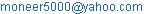(M. A. S. Saeed)

Abdelilah K. Hassan Sedeeg, Mohand M. Abdelrahim Mahgoub, Muneer A. Saif Saeed. An Application of the New Integral "Aboodh Transform" in Cryptography. Pure and Applied Mathematics Journal. Vol. 5, No. 5, 2016, pp. 151-154. doi: 10.11648/j.pamj.20160505.12

Received: August 7, 2016; Accepted: August 23, 2016; Published: September 9, 2016

Abstract: Cryptography is the science of providing security for information, It has been used historically as a means of providing secure communication between individuals. Message encryption has become very essential to avoid the threat against possible attacks by hackers during transmission process of the message. In this paper authors have proposed a method of cryptography, in which authors have used Aboodh transform for encrypting the plain text and corresponding inverse Aboodh transform for decryption.

Keywords: Cryptography, Encryption, Decryption, Aboodh Transform

Contents

1. Introduction

Cryptography, the mathematics of encryption, plays an indispensable part in numerous fields, and a vast range of daily activities, such as electronic commerce, bank card payments and electronic building and so on. Cryptography is the only most important tool that avoids the threat against possible attacks by hackers during transmission process of the message, It is one of the cornerstones of Internet security. Cryptography is the only most important tool that avoids the threat against possible attacks by hackers during transmission process of the message.

Cryptography [5-10] referred almost exclusively to encryption, which is the process of converting ordinary information (called plaintext) into unintelligible text (called cipher text). Decryption is the reverse, in other words, moving from the unintelligible cipher text back to plaintext. A cipher (or cipher) is a pair of algorithms that create the encryption and the reversing decryption. The detailed operation of a cipher is controlled both by the algorithm and in each instance by a "key". The key is a secret (ideally known only to the communicants), usually a short string of characters, which is needed to decrypt the cipher text. (Fig. 1) [11-15].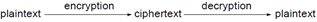Fig. 1. Basic encryption and Decryption.

2. Proposed Technique

In the present paper a new cryptographic scheme is proposed using Aboodh Transform [1-4]. Aboodh transform is used for encrypting the plain text and corresponding inverse Aboodh transform is used for decryption. Aboodh transform was introduced by Khalid Aboodh in 2013. Aboodh transform is a widely used integral transform in mathematics and electrical engineering that transforms a function of time into a function of complex frequency. The inverse Aboodh transform takes a complex frequency domain function and yields a function defined in the time domain. Proposed algorithm provides as many transformations as per the requirements which are the most useful factor for changing key. Therefore it is very difficult for an eyedropper to trace the key by any attack. The implementation has been done in visual basic (VB).

3. Aboodh Transform

Definition: Consider functions in the set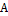defined by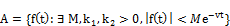For a given function in the set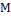must be finite number,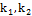may be finite or infinite. Aboodh transform denotedby the operator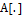is defined by the integral equation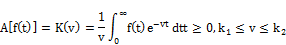Properties of Aboodh transform:-

Linearity:-Aboodh transform is a linear transformation which means that the transform of a sum of waveforms is the sum of their transforms. Stated formally the linearity property is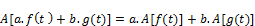Where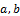are constants.

The above result can easily be generalized to more than two functions.

Aboodh transformation &Inverse Aboodh Transform of some elementary functions:-

Elementary functions include algebraic and transcendental functions.

1.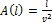, whereis constant.

2.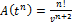3.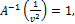4.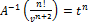4. Proposed Methodology

The following algorithm provides an insight into the proposed cryptographic scheme. The sender converts the original message or plain text into cipher text using the following steps.

4.1. Encryption Algorithm

I)       Treat every letter in the plain text message as a number, so that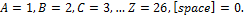II)    The plain text message is organized as finite sequence of numbers based on the above conversion. For example our text is "TEACHERS". Based on the above step; we know that,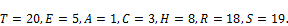Therefore our plain text finite sequence is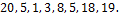III)  If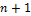is the number of term in the sequence; consider a polynomial of degree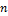with coefficient as the term of the given finite sequence. Above finite sequence containsterms. Hence consider a polynomial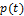of degree.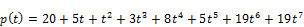Take Aboodh transform of polynomial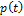.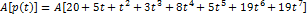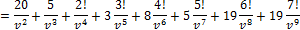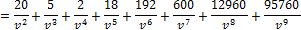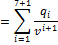Next find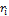such that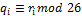for each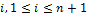. Therefore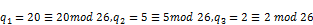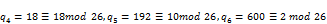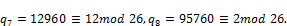IV)  Hence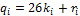. Thus we get a key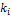for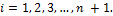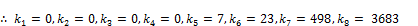V)     Now consider a new finite sequence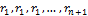i.e.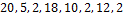.

4.2. Decryption Algorithm

I) Consider the cipher text and key received from sender. In the above example cipher text is "TEBRJBLB" and key is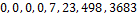.

II) Convert the given cipher text to corresponding finite sequence of numbers,.

III) Let,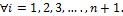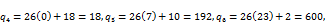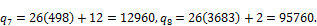IV)    Let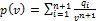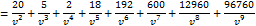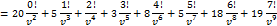V)      Now take the Inverse Aboodh transform of p(v).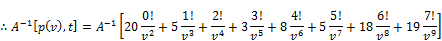VI)    Consider the coefficient of a polynomialas a finite sequence.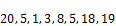.

VII) Now translating the number of above finite sequence to alphabets. We get the original plain text as "TEACHERS".

5. Implementation of the Efficient Algorithm

Visual basic programming language is one of the most widely use high level language today because of its advantages . In this parta program has been written in visual basic language (VB), forthe implementation of the Encryption Algorithms and Implementation Decryption Algorithms in section 4.

5.1. Implementation Encryption Algorithms

Private Sub Enc_btn_Click()

Dim no_of_ltr As Integer

Dim ltrs, e(26) As String

no_of_ltr = Len(Me.text1)

Dim p(26), ris(1000), g, qis(26) As Long

Me.enc = "" Me.dcr = ""Me.mdd = ""Me.py = ""Me.dcr = ""Me.keys = ""Me.enc_txt = ""e(1) = "A"e(2) = "B"e(3) = "C"e(4) = "D"e(5) = "E"e(6) = "F"e(7) = "G"e(8) = "H"e(9) = "I"e(10) = "J"e(11) = "K"e(12) = "L"e(13) = "M"e(14) = "N"e(15) = "O"e(16) = "P"e(17) = "Q"e(18) = "R"e(19) = "S"e(20) = "T"e(21) = "U"e(22) = "V"e(23) = "W"e(24) = "X"e(25) = "Y"e(26) = "Z"

For i = 1 Tono_of_ltr

ltrs = Mid(Me.text1, (i), 1)

'MsgBox (ltrs)

For j = 1 To 26

If ltrs = e(j) Then

'MsgBox (i)

'MsgBox (j)

'MsgBoxe(j)

qis(i) = j * Factorial(i - 1)

ris(i) = qis(i) Mod 26

Me.enc = Me.enc&qis(i) & "-"

Me.mdd = Me.mdd&ris(i) & "-"

ks = ((qis(i) - ris(i)) / 26)

Me.keys = Me.keys&ks& "-"

Me.enc_txt = Me.enc_txt&e(ris(i))

End If

Next

'MsgBox (qis(i))

'LArray(i) = ltrs

'Me.enc = Me.enc& g & "-"

'Me.mdd = Me.mdd&ris(i) & "-"

'Me.dcr = Me.dcr&Chr(g)

Next

End Sub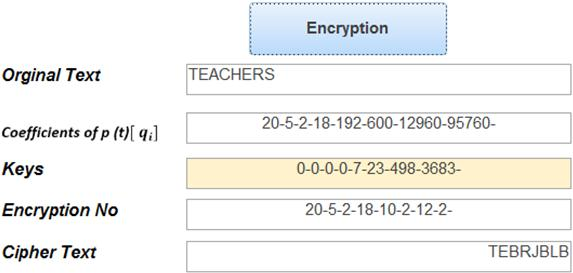Fig. 2. Implementation Encryption.

5.2. Implementation Decryption Algorithms

Private Sub Dec_Btn_Click()

Dim pos, intCount As Integer

Dim LArray(1000), ky(26), e(26), strTest, d, strArray(), ee(1000) As String

Dim p(26), qis(1000), no(1000), ris(26), key(26) As Long

'LArray = Split(Me.text1)

pos = Len(Me.text1)

'Me.key = ""Me.dcr = ""Me.mdd = ""Me.py = ""Me.dcr = ""Me.encrpt = ""e(1) = "A"e(2) = "B"e(3) = "C"e(4) = "D"e(5) = "E"e(6) = "F"e(7) = "G"e(8) = "H"e(9) = "I"e(10) = "J"e(11) = "K"e(12) = "L"e(13) = "M"e(14) = "N"e(15) = "O"e(16) = "P"e(17) = "Q"e(18) = "R"e(19) = "S"e(20) = "T"e(21) = "U"e(22) = "V"e(23) = "W"e(24) = "X"e(25) = "Y"e(26) = "Z"

strTest = Me.keys

strArray = Split(strTest, "-")

For intCount = LBound(strArray) To UBound(strArray) - 1

key(intCount) = (Trim(strArray(intCount)))

' MsgBox (intCount)

Next

For i = 1 Topos

d = Mid(Me.text1, (i), 1)

For j = 1 To 26

If d = e(j) Then

ris(i) = j ' * Factorial(i - 1)

'qis(i) = ris(i) Mod 26

'Me.enc = Me.enc&ris(i) & "-"

'Me.mdd = Me.mdd&qis(i) & "-"

Me.py = Me.py &ris(i) & "-"

qis(i) = ris(i) + (key(i - 1) * 26)

Me.mdd = Me.mdd&qis(i) & "-"

no(i) = (qis(i) / Factorial(i - 1))

org_txt = org_txt&e(no(i))

Me.encrpt = Me.encrpt&no(i) & "-"

'MsgBox ((ris(i))) ' - qis(i)) / 26)

End If

Next

'MsgBox (ris(i))

'LArray(i) = d

'g = Asc(d)

'p(i) = g

'qis(i) = p(i) Mod 26

'Me.py = Me.py &p(i) & "-"

'Me.enc = Me.enc& g & "-"

'Me.mdd = Me.mdd&qis(i) & "-"

'ee(i) = Chr(p(i))

'Me.encrpt = Me.encrpt&ee(i) & "-"

'Me.dcr = Me.dcr&Chr(g)

Next

End Sub

Public Function Factorial(ByVal X As Long) As Long

If X <= 1 Then

Factorial = 1

Else

Factorial = X * Factorial(X - 1)

End If

End Function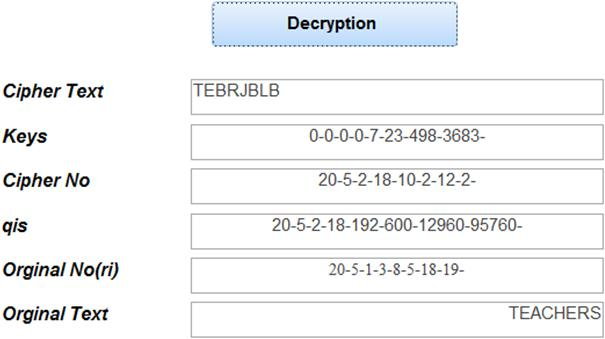Fig. 3. Implementation Decryption.

6. Conclusion

Computers are good at doing mathematics. When we create a system to translate some piece of information into numbers (such as we do with text and ordinals or with space and coordinate systems), computer programs can process these numbers quickly and efficiently. Cryptography is one of the first lines of defense against hackers and crackers in today's world. Thus, it will stay important for a long time to come. In the proposed work a new cryptographic scheme is introduced using Aboodh Transform and the private key is the number of multiples of mod n. Visual Basic is more convenient to use in the Cryptography and is less prone to errors.

Acknowledgement

Author is thankful to Mr. Abdullah Musa, Mr. Hassan Mohammed, Mr. Khider Yousif and Ms. Nan Mohammed, for support to this work.

References

1. K. S. Aboodh, The New Integral Transform "Aboodh Transform" Global Journal of pure and Applied Mathematics, 9 (1), 35-43 (2013).
2. K. S. Aboodh, Application of New Transform "Aboodh transform" to Partial Differential Equations, Global Journal of pure and Applied Math, 10 (2), 249-254 (2014).
3. Khalid SulimanAboodh, Homotopy Perturbation Method and Aboodh Transform for Solving Nonlinear Partial Differential Equations, Pure and Applied Mathematics JournalVolume 4, Issue 5, October 2015, Pages: 219-224 .
4. Khalid SulimanAboodh, Solving Fourth Order Parabolic PDE with Variable Coefficients Using Aboodh Transform Homotopy Perturbation Method, Pure and Applied Mathematics Journal 2015; 4 (5): 219-224 .
5. A. P. Hiwarekar "A NEW METHOD OF CRYPTOGRAPHY USING LAPLACE TRANSFORM"International Journal of Mathematical Archive-3 (3), 2012, Page: 1193-1197 .
6. A. P. Stakhov, "The golden matrices and a new kind of cryptography", Chaos, Soltions and Fractals .
7. Stallings W., Cryptography and Network Security, Fourth Edition, Prentice Hall, 2005 .
8. Grewal B. S. – Higher Engineering Mathematics, Khanna Pub. Delhi, 2005.
9. Barr T. H., Invitation to Cryptography, Prentice Hall, (2002).
10. Blakley G. R., Twenty years of Cryptography in the open literature, Security and Privacy 1999, Proceedingsof the IEEE Symposium, 9-12, May 1999 .
11. Petersen K. – Notes on Number Theory and Cryptography, http://www.math.unc.edu/ Faculty petersen/ Coding/cr2.pdf.
12. Overbey J. - Traves W. and Wojdylo J. – On the Key space of the Hill Cipher, Cryptologia, 29 (1), January 2005, 59-72.
13. A. P. Hiwarekar, Application of Laplace Transform For Cryptographic Scheme, Proceedings of the World Congress on Engineering 2013 Vol I, WCE 2013, July 3 - 5, 2013, London, U.K.
14. Swati Dhingra, Archana A. Savalgi, Swati Jain, Laplace Transformation based Cryptographic Technique in Network Security,International Journal of Computer Applications (0975 – 8887) Volume 136 – No.7, February 2016.
15. A. P. Hiwarekar, A NEW METHOD OF CRYPTOGRAPHY USING LAPLACE TRANSFORM, International Journal of Mathematical Archive-3 (3), 2012, Page: 1193-1197.
16. Abdulkadir Baba HASSAN, Matthew Sunday ABOLARIN, Onawola Hassan JIMOH, The Application of Visual Basic Computer Programming Language to Simulate Numerical Iterations, Leonardo Journal of Sciences, Issue 9, July-December 2006 p. 125-136.

 Contents 1. 2. 3. 4. 4.1. 4.2. 5. 5.1. 5.2. 6.
Article ToolsAbstractPDF(401K)